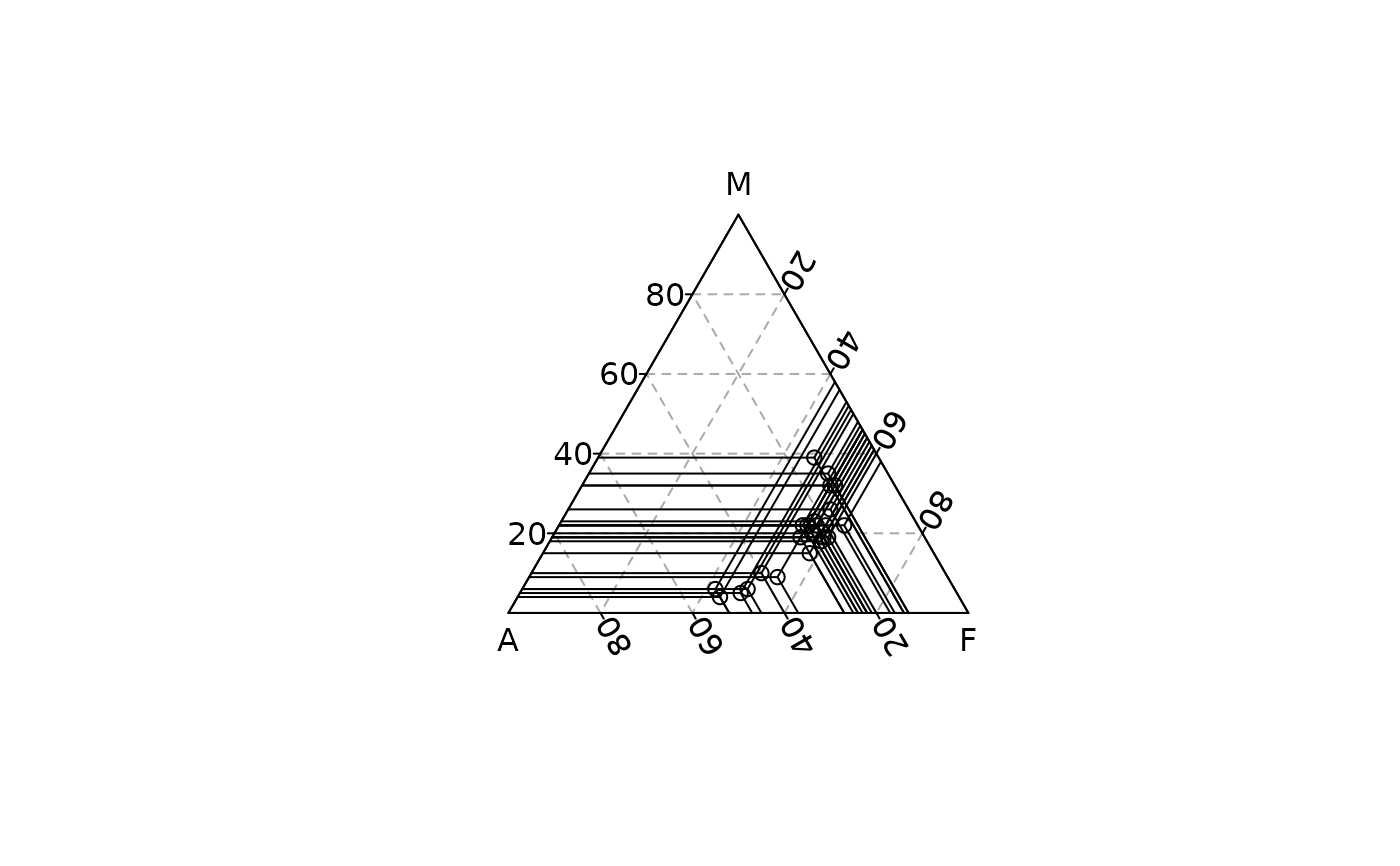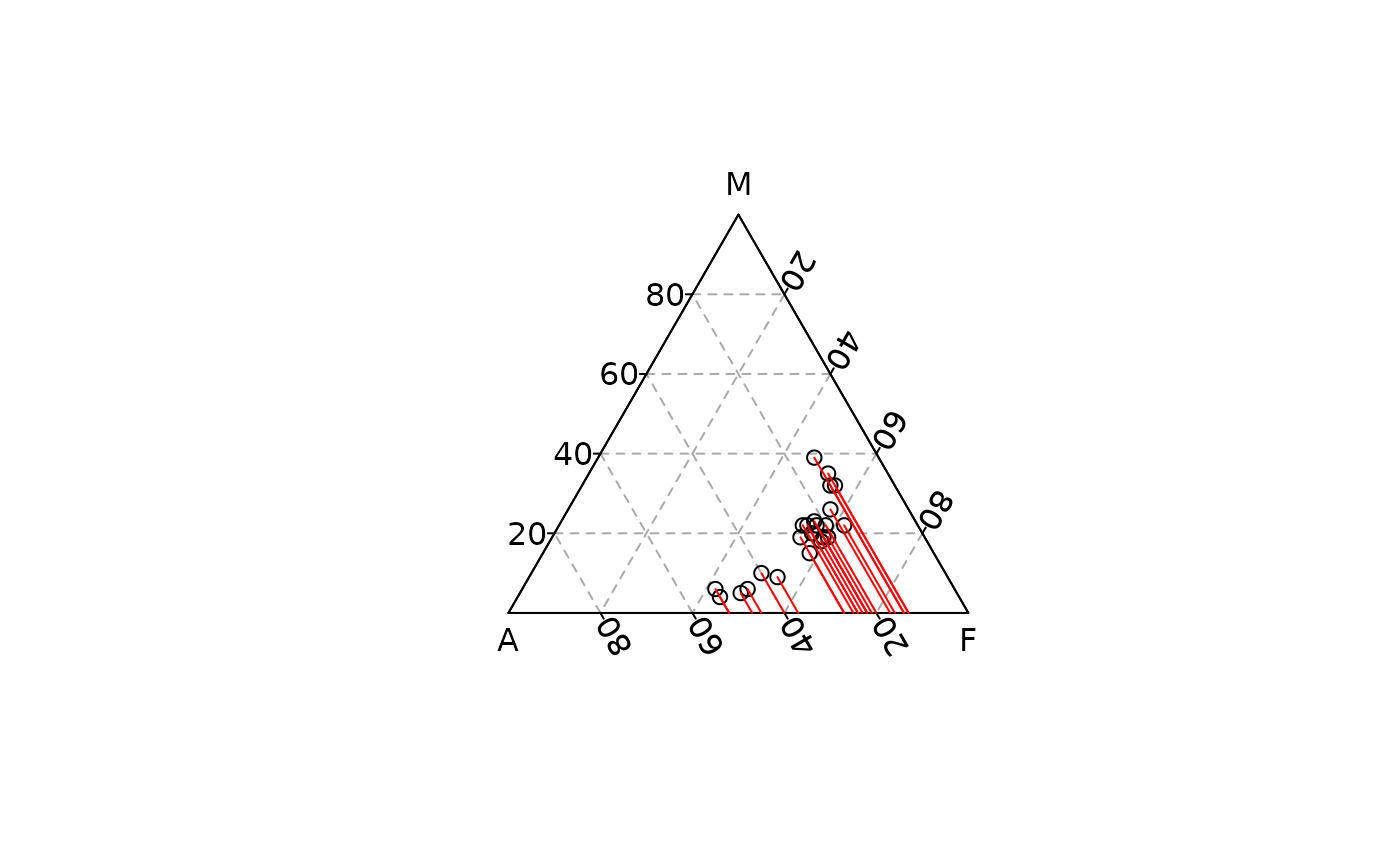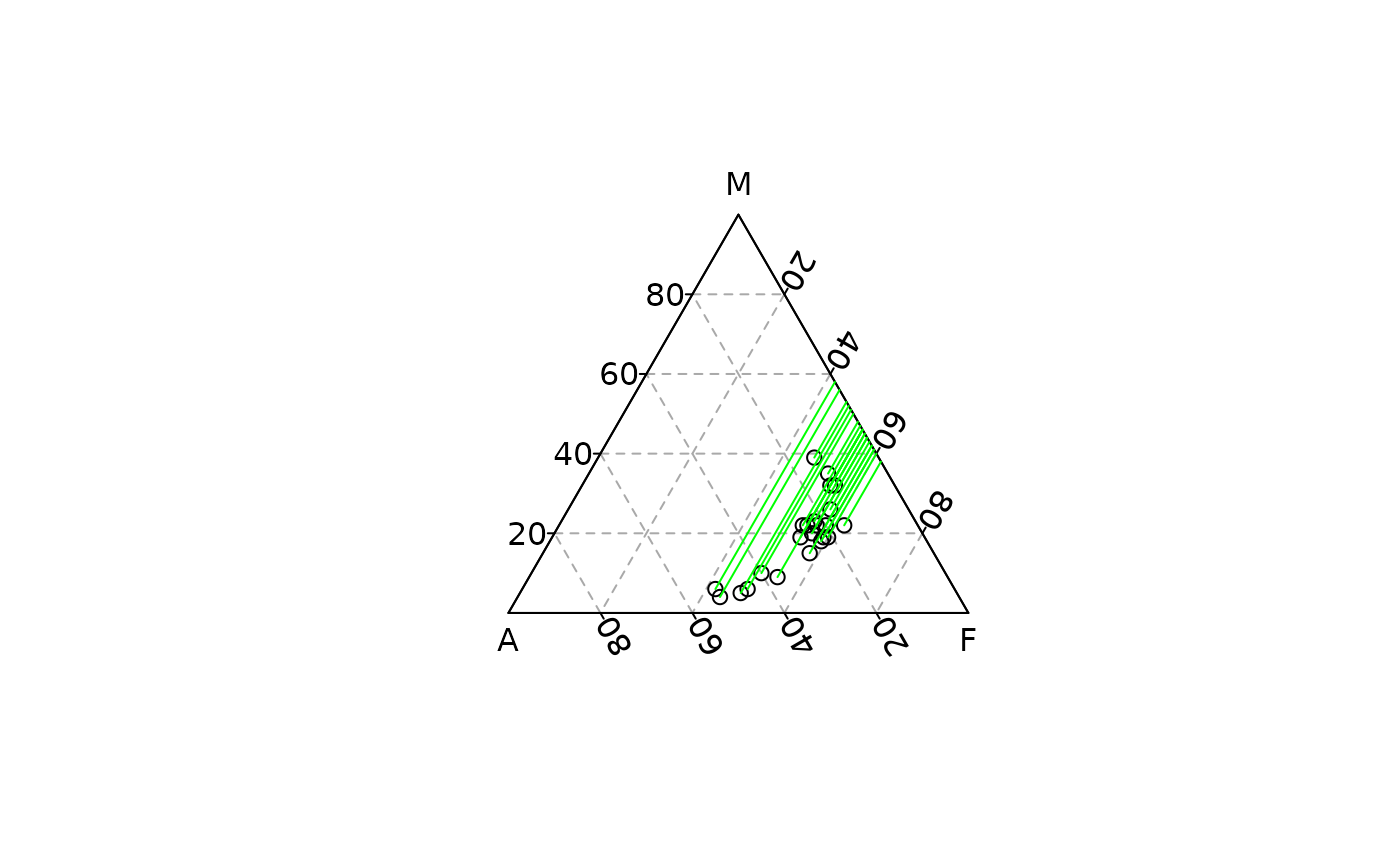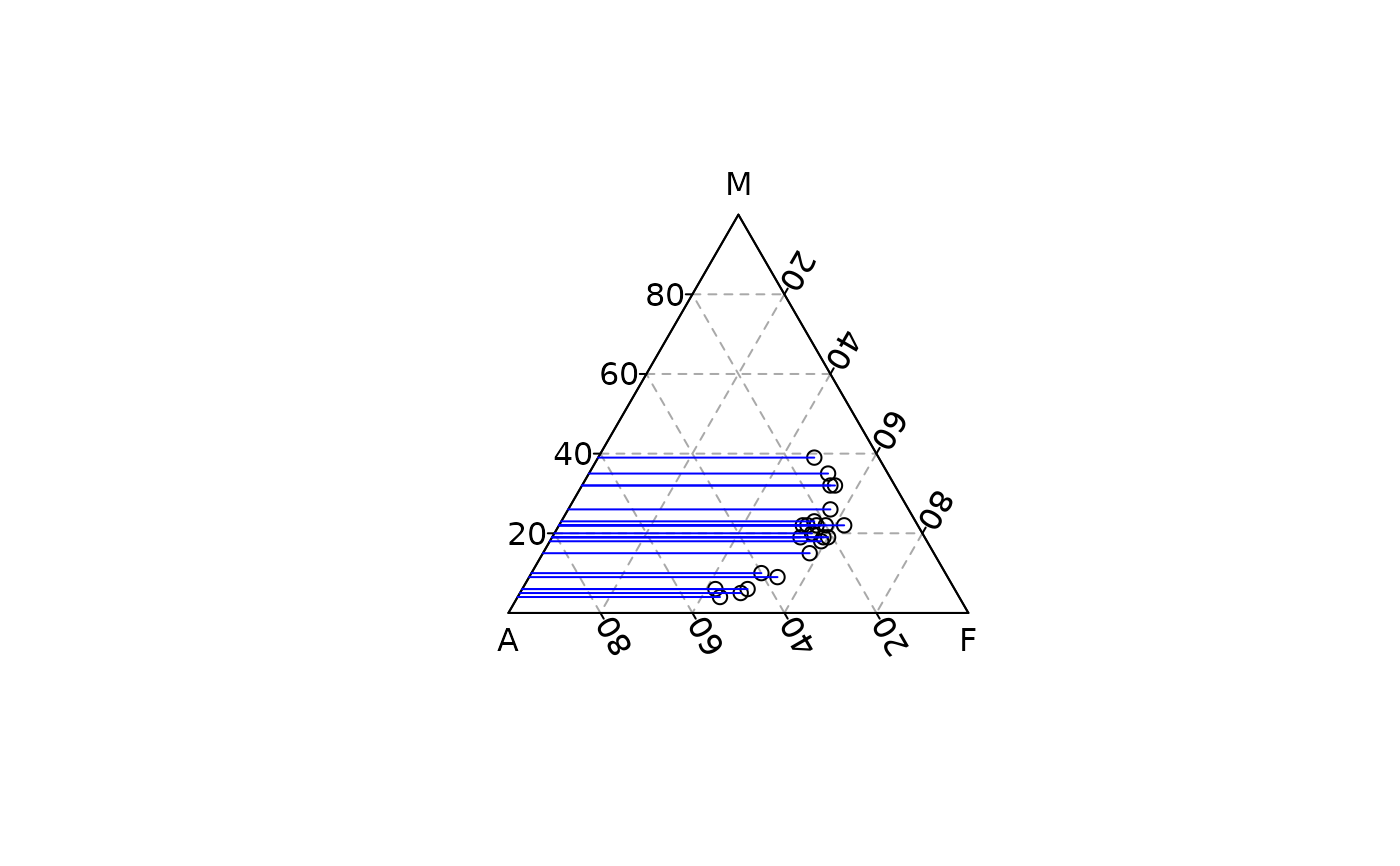Draw lines that intersect at a point.

## Usage

ternary_crosshairs(x, y, z, ...)

# S4 method for numeric,numeric,numeric
ternary_crosshairs(x, y, z, x_mark = TRUE, y_mark = TRUE, z_mark = TRUE, ...)

# S4 method for ANY,missing,missing
ternary_crosshairs(x, x_mark = TRUE, y_mark = TRUE, z_mark = TRUE, ...)

## Arguments

x, y, z

A numeric vector giving the x, y and z ternary coordinates of a set of points. If y and z are missing, an attempt is made to interpret x in a suitable way (see grDevices::xyz.coords()).

...

Further graphical parameters (see graphics::par()) may also be supplied as arguments, particularly, line type, lty, line width, lwd and color, col. Also the line characteristics lend, ljoin and lmitre.

x_mark, y_mark, z_mark

A logical scalar: should the x, y or z axis component be drawn?

## Value

ternary_crosshairs() is called it for its side-effects.

Other geometries: ternary_arrows(), ternary_lines(), ternary_points(), ternary_polygon(), ternary_segments(), ternary_text()

N. Frerebeau

## Examples

## Add cross-hairs
## Data from Aitchison 1986
ternary_plot(lava, panel.first = ternary_grid())
ternary_crosshairs(lava)ternary_plot(lava, panel.first = ternary_grid())
ternary_crosshairs(lava, y_mark = FALSE, z_mark = FALSE, col = "red")ternary_plot(lava, panel.first = ternary_grid())
ternary_crosshairs(lava, x_mark = FALSE, z_mark = FALSE, col = "green")ternary_plot(lava, panel.first = ternary_grid())
ternary_crosshairs(lava, x_mark = FALSE, y_mark = FALSE, col = "blue")